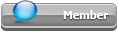## Featured Articles

Check out the latest featured articles.## File Library2# Multithreading App With Python And Prode Properties

python prode fluid properties multithreading parallel

3 replies to this topic
|

### #1Alpe

Alpe

Junior Member

•• Members
•• 11 posts

Posted 13 June 2021 - 02:01 AM

I wrote a Python procedure which adopts heuristic optimization methods and takes much time to complete,

how can I run multiple threads in parallel with Python and Prode ?

Can you provide an example ?

### #2PaoloPemi

PaoloPemi

Gold Member

•• Members
•• 504 posts

Posted 13 June 2021 - 02:43 AM

Hi Alpe,

I confirm (see below the examples) that you can run multiple tasks in parallel with Prode and Python ,

for multithreading in Python you can import threading library while Prode doesn't require any specific settings,

you would need a multicore cpu , my cpu has four cores and I can execute my apps about four times faster with multithreading.

Another possibility is to execute on a cloud, some providers offer multicore cpus at reasonable prices, you can install Prode on a Linux server, see these examples

https://www.prode.co...gramcompare.php

https://www.prode.co...ies/hydrate.php

herebelow the examples of Python and Prode Properties running in single and multiple threads,  reported results are for a Intel i7 3740QM cpu (four cores)

results in Python 3.8 (64-bit) console

Total time (seconds) -> 0.16275644302368164
245.0   K   214036.75   Pa.a
250.0   K   297396.875   Pa.a
255.0   K   402031.21875   Pa.a
260.0   K   533987.5   Pa.a
265.0   K   653298.9375   Pa.a
270.0   K   760583.0   Pa.a
275.0   K   1075351.875   Pa.a
280.0   K   1814863.25   Pa.a
285.0   K   3070172.75   Pa.a
290.0   K   5376445.5   Pa.a
Total time (seconds) -> 0.04987978935241699
245.0   K   214036.75   Pa.a
250.0   K   297396.875   Pa.a
255.0   K   402031.21875   Pa.a
260.0   K   533987.5   Pa.a
265.0   K   653298.9375   Pa.a
270.0   K   760583.0   Pa.a
275.0   K   1075351.875   Pa.a
280.0   K   1814863.25   Pa.a
285.0   K   3070172.75   Pa.a
290.0   K   5376445.5   Pa.a

# hydrate formation conditions
# calculated with Prode Properties

import time
import prode
from array import array

# global vars
pt = array('f',[0,0,0,0,0,0,0,0,0,0,0])
pp = array('f',[0,0,0,0,0,0,0,0,0,0,0])
stream = 1

# define a function to calculate hydrate formation conditions
def hydform(i1,t):
# convert t (unit K) to user unit
tt = prode.umcr(t,17,1)
pt[i1] = tt
# call Prode Properties to calculate hydrate pressure
# mode 2 instructs Prode to evaluate only structures I (as in original work)
pp[i1] = prode.hydp(stream,tt,2)

# for a few formers, obtain internal codes from CAS numbers (or chemical formula, names etc.)
C1 = prode.idc(74828)
C2 = prode.idc(74840)
C3 = prode.idc(74986)
IC4= prode.idc(75285)
NC4= prode.idc(106978)
H2S= prode.idc(7783064)
N2 = prode.idc(7727379)
CO2= prode.idc(124389)
H2O= prode.idc(7732185)

# define a stream with hydrate formers plus water
prode.inits(stream)
prode.putsm(stream,1,0,115) # fugacity, vapor phase, PRCPANHV model
prode.putsm(stream,1,1,115) # fugacity, liquid phase, PRCPANHV model
prode.putsm(stream,1,2,151) # fugacity, solid phase, SPRCPANHV model
prode.putsm(stream,1,3,170) # fugacity, hydrate phase, HPRCPANHV model
prode.putscc(stream,1,C1)   # mixture evaluated by Robinson, 1966
prode.putsz(stream,1,0.808)
prode.putscc(stream,2,CO2)
prode.putsz(stream,2,0.13)
prode.putscc(stream,3,H2S)
prode.putsz(stream,3,0.06)
prode.putscc(stream,4,H2O)
prode.putsz(stream,4,0.06)
prode.sets(stream)

# call hydform ten times in range 245 K to 290 K
start = time.time()
hydform(1,245.0)
hydform(2,250.0)
hydform(3,255.0)
hydform(4,260.0)
hydform(5,265.0)
hydform(6,270.0)
hydform(7,275.0)
hydform(8,280.0)
hydform(9,285.0)
hydform(10,290.0)
end = time.time()

print('Total time (seconds) ->',end-start,'\r')
for i in range(1,11):
print(pt[i],' ',prode.sums(17),' ',pp[i],' ',prode.sums(15),'\r')

# hydrate formation conditions
# calculated with Prode Properties

import time
import prode
from array import array

# global vars
pt = array('f',[0,0,0,0,0,0,0,0,0,0,0])
pp = array('f',[0,0,0,0,0,0,0,0,0,0,0])
stream = 1

# define a function to calculate hydrate formation conditions
def hydform(i1,t):
# convert t (unit K) to user unit
tt = prode.umcr(t,17,1)
# call Prode Properties to calculate hydrate pressure
# mode 2 instructs Prode to evaluate only structures I (as in original work)
p = prode.hydp(stream,tt,2)
llock.acquire()
pt[i1] = tt
pp[i1] = p
llock.release()

# for a few formers, obtain internal codes from CAS numbers (or chemical formula, names etc.)
C1 = prode.idc(74828)
C2 = prode.idc(74840)
C3 = prode.idc(74986)
IC4= prode.idc(75285)
NC4= prode.idc(106978)
H2S= prode.idc(7783064)
N2 = prode.idc(7727379)
CO2= prode.idc(124389)
H2O= prode.idc(7732185)

# define a stream with hydrate formers plus water
prode.inits(stream)
prode.putsm(stream,1,0,115) # fugacity, vapor phase, PRCPANHV model
prode.putsm(stream,1,1,115) # fugacity, liquid phase, PRCPANHV model
prode.putsm(stream,1,2,151) # fugacity, solid phase, SPRCPANHV model
prode.putsm(stream,1,3,170) # fugacity, hydrate phase, HPRCPANHV model
prode.putscc(stream,1,C1)   # mixture evaluated by Robinson, 1966
prode.putsz(stream,1,0.808)
prode.putscc(stream,2,CO2)
prode.putsz(stream,2,0.13)
prode.putscc(stream,3,H2S)
prode.putsz(stream,3,0.06)
prode.putscc(stream,4,H2O)
prode.putsz(stream,4,0.06)
prode.sets(stream)

# run 10 indipendent threads to calculate 10 points
# ranging from 245 K to 290 K

start = time.time()
t1.start()
t2.start()
t3.start()
t4.start()
t5.start()
t6.start()
t7.start()
t8.start()
t9.start()
t10.start()

t1.join()
t2.join()
t3.join()
t4.join()
t5.join()
t6.join()
t7.join()
t8.join()
t9.join()
t10.join()

end = time.time()

print('Total time (seconds) ->',end-start,'\r')
for i in range(1,11):
print(pt[i],' ',prode.sums(17),' ',pp[i],' ',prode.sums(15),'\r')

### #3Alpe

Alpe

Junior Member

•• Members
•• 11 posts

Posted 13 June 2021 - 02:46 AM

### #4mgerian

mgerian

Brand New Member

•• Members
•• 6 posts

Posted 14 June 2021 - 12:11 AM

thanks for the links to free online calc's Paolo !

I have found these pages very useful !

### Similar Topics